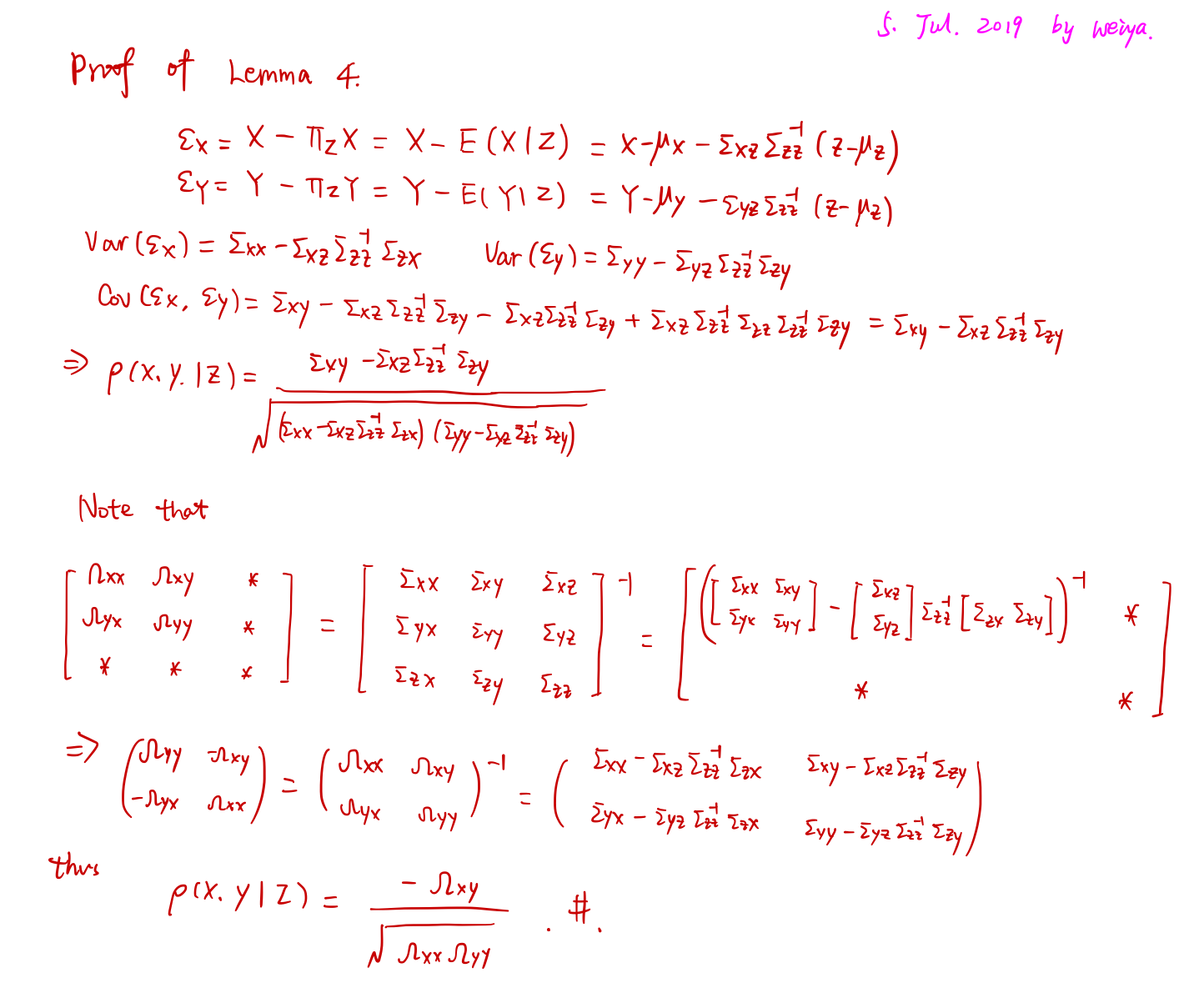# MLE for MTP2

##### Posted on Jul 05, 2019 (Update: Jul 25, 2019)
Tags: MLE, Graph

A $p$-variate real-valued distribution with density $f$ w.r.t. a product measure $\mu$ is Multivariate totally positive of order 2 (MTP2) if the density satisfies

$f(x)f(y)\le f(x\land y)f(\lor y)\,.$

$N(\mu, \Sigma) is MTP2 iff$K:=\Sigma^{-1}$is a symmetric$M$-matrix, i.e.,$K_{ij}\le 0$for all$i\neq j$, or equivalently, if all partial correlations are nonnegativeby the following lemma. ### Partial Correlation Graphs Let$X, Y\in\bbR$and$Z$be a random vector. The partial correlation between$X$and$Y$, given$Z$is a measure of association between$X$and$Y$after removing the effect of$Z$. Specifically,$\rho(X,Y\mid Z)$is the correlation between$\epsilon_X$and$\epsilon_Y$where $\epsilon_X = X-\Pi_ZX,\quad \epsilon_Y=Y-\Pi_ZY\,.$ Here,$\Pi_ZX$is the projection of$X$onto the linear space spanned by$Z$. That is,$\Pi_ZX=\bbE[X\mid Z]$. For a graph, let$X=(X(1),\ldots,X(d))$and let$\rho_{jk}$denote the partial correlation between$X(j)$and$X(k)$given all the other variables. Let$R={\rho_{jk}}$be the$d\times d$matrix of partial correlations. The matrix$R$is given by$R(j,k)=-\Omega_{jk}/\sqrt{\Omega_{jj}\Omega_{kk}}$where$\Omega=\Sigma^{-1}$and$\Sigma$is the covariance matrix of$X$.### Literatures on MTP2 Gaussian graphical model • form a sub-class of non-frustrated Gaussian graphical model • efficient structure estimation algorithms based on thresholding covariances after conditioning on subsets of variables of limited size • Slawski and Hein: efficient learning procedures based on convex optimization. The paper is closely related to this approach. ### Task Given$n$iid samples from$N(x,\Sigma)$, where$\Sigma$is unknown with size$p\times p$. W.L.O.G, assume$\mu=0$and focus on the estimation of$\Sigma$. Let$S$be the sample covariance matrix based on$n$samples. Then the log-likelihood function is, up to additive and multiplicative constants, given by $\ell(K; S) = \log\det K-\tr(SK)\,.$ Notations: •$\bbS^p$: the cone (?) of real symmetric matrices of size$p\times p$•$\bbS^p_{\succ 0}$: the positive definite elements •$\bbS^p_{\succeq 0}$: the positive semidefinite elements Since$M$-matrices form a convex subset of$\bbS^p_{\succ 0}$, the optimization problem for computing the MLE for MTP2 Gaussian models is a convex optimization problem. Slawski and Hein: • show that the MLE exists when$n\ge 2$• provide empirical evidence that the MTP2 constraint serves as an implicit regularizer and leads to sparsity in$K$. The paper: • analyze the sparsity pattern of the MLE$\hat K$under the MTP2 constraint. • obtain a simple upper bound for the ML graph$G(\hat K)\$.

Published in categories Note